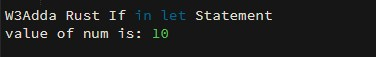# Rust If in Let Statement

In this tutorial you will learn about the Rust If in Let Statement and its application with practical example.

## Rust If Let Statement

Rust If Let Statement is a combination of if and let statements in a more concise way, where value of if statement is assigned to let statement.

Syntax:-

Here, if Condition is a Boolean expression that results in either True or False, if it results in True then statements inside if body are executed and result value is assigned to var_name, if it results in False then statements inside else body are executed and result value is assigned to var_name.

Example :-

Output :-In this tutorial we have learn about the Rust If in Let Statement and its application with practical example. I hope you will like this tutorial.